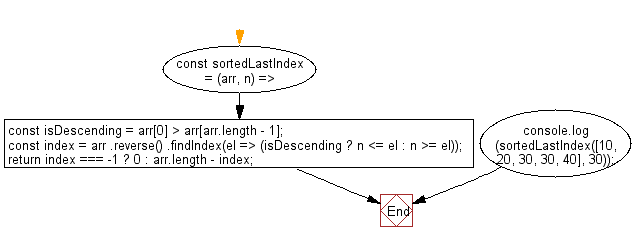# JavaScript Sorting Algorithm: Sorted last index

## JavaScript Sorting Algorithm: Exercise-23 with Solution

Write a JavaScript program to find the highest index at which a value should be inserted into an array to maintain its sort order.

• Loosely check if the array is sorted in descending order.
• Use Array.prototype.reverse() and Array.prototype.findIndex() to find the appropriate last index where the element should be inserted.

Sample Solution:

JavaScript Code:

``````const sortedLastIndex = (arr, n) => {
const isDescending = arr > arr[arr.length - 1];
const index = arr
.reverse()
.findIndex(el => (isDescending ? n <= el : n >= el));
return index === -1 ? 0 : arr.length - index;
};
console.log(sortedLastIndex([10, 20, 30, 30, 40], 30));
```
```

Sample Output:

```4
```

Flowchart:Live Demo:

See the Pen javascript-common-editor by w3resource (@w3resource) on CodePen.

Improve this sample solution and post your code through Disqus

What is the difficulty level of this exercise?

Test your Programming skills with w3resource's quiz.

﻿